# Python - Select Multiple Checkbox Choices to Calculate a Field

5911
5
06-15-2016 01:29 PMNew Contributor III

Hello all,

I want to allow the user who runs the script to select however many choices from a list to populate a field.

The choices are all string values I created in the script tool parameters using the Value List Filter for the Expression parameter.

For example:

After using Add Field function, I need to populate/calculate the "Choices" attribute column.

*The Choices field is not present before running the script. This is just to help visualize what I need.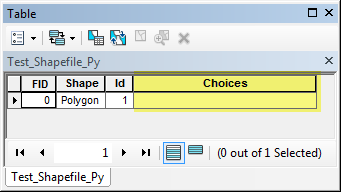The script window.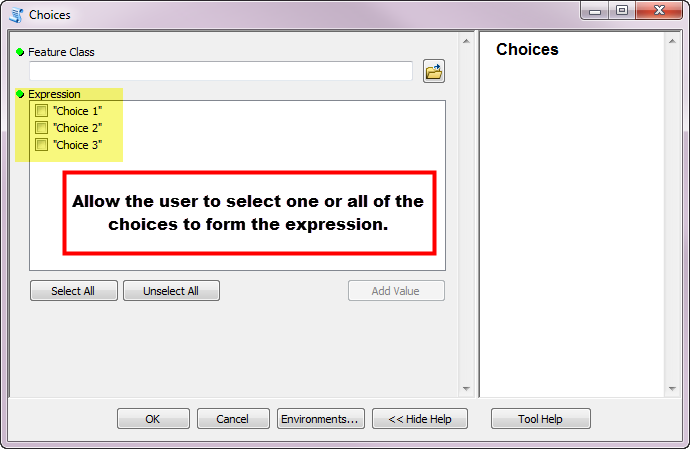The following is the code for this script.

*This was extracted from Model Builder.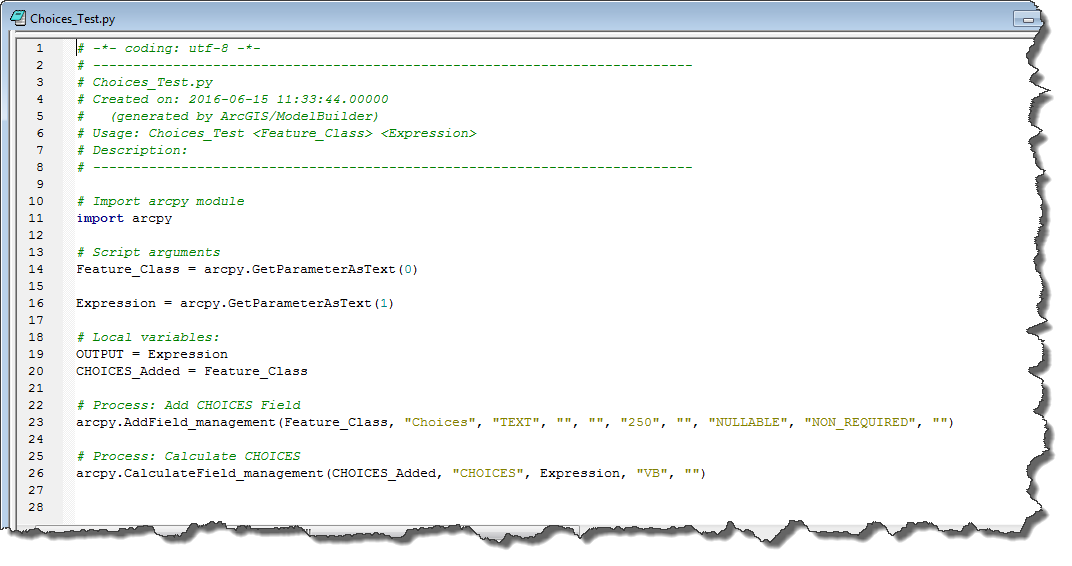The following is the validation code for the script (which is the standard for a new script).The following shows the parameters for the script.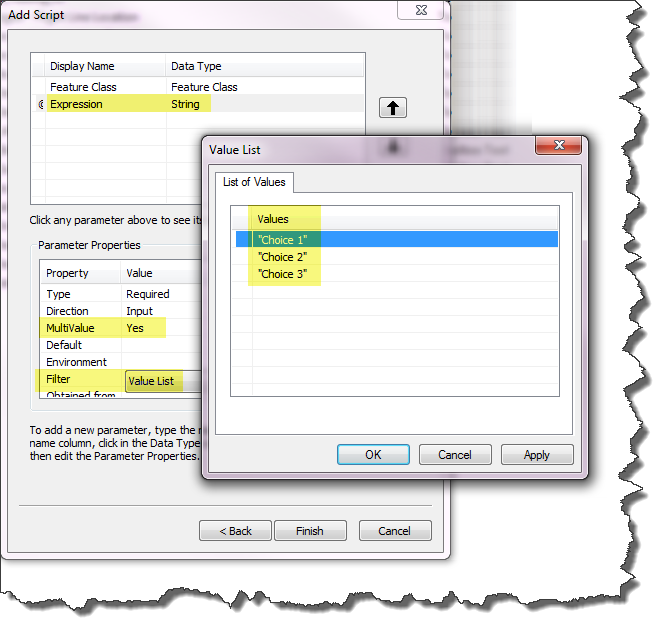The following is the error message I get when running the script.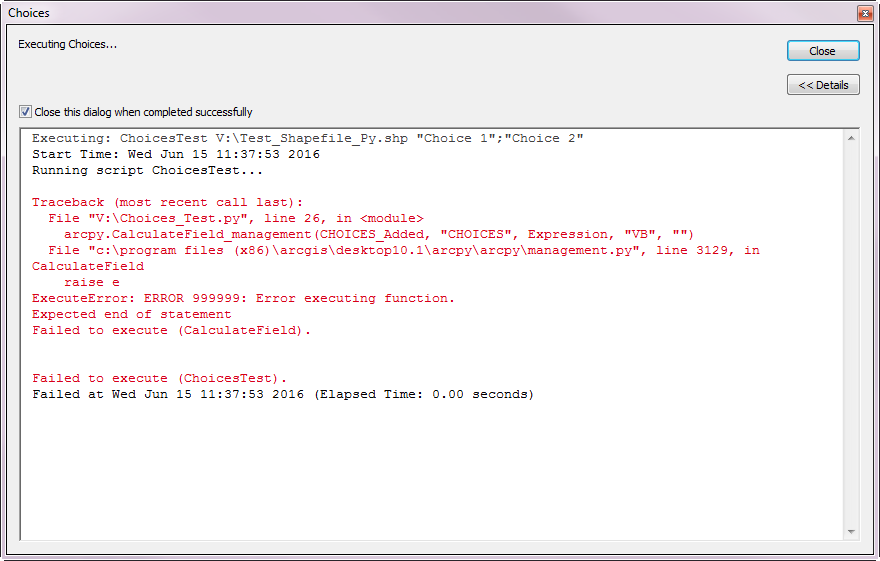I am new to Python and could use some guidance.

Thanks,

Sarah

5 RepliesbyMVP Esteemed Contributor

I would confirm the field syntax since vb requires [ ] around the field name and python the ! ! marks.  And while you are at it, I would switch to python to give your development some future life.

As a test, do a manual Calculate Field—Help | ArcGIS for Desktop

then check the Results window to get the correct format just to make surebyMVP Regular Contributor

You could also add the choices as Boolean parameters, then in you script check which choices are "true" and construct a string from the values to use in your CalculateField expression.New Contributor III

Since posting this, I have revised my code but the script is still not working completely.

The field i'm trying to populate from the checkbox choices won't populate even though the script says it ran correctly.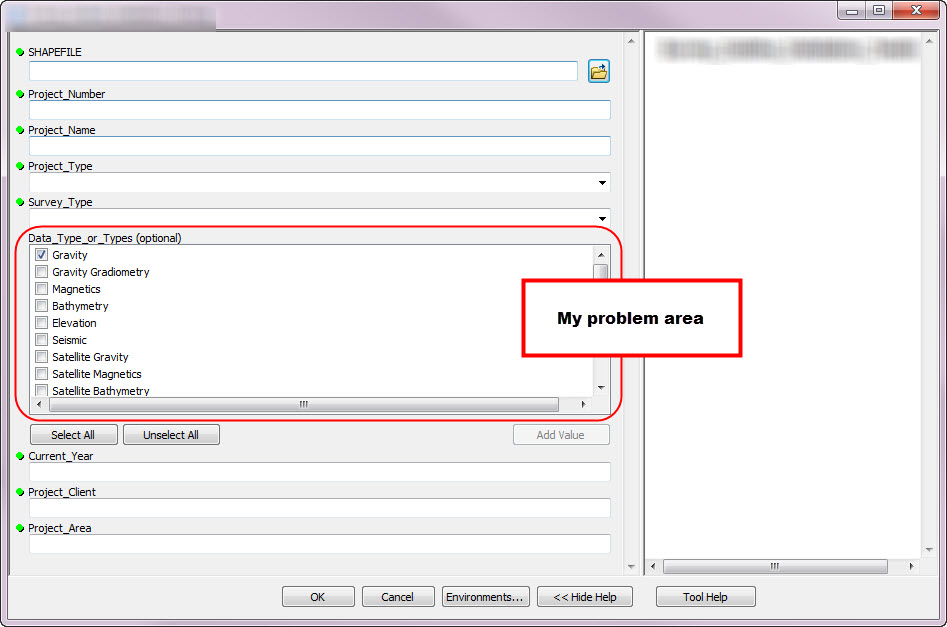I've provided both my Script and the Validation code I'm using.

My Script:

# Import arcpy modules
import arcpy, os

# Variables
SHAPEFILE = arcpy.GetParameterAsText(0)

Project_Number = arcpy.GetParameterAsText(1)

Project_Name = arcpy.GetParameterAsText(2)

Project_Type = arcpy.GetParameterAsText(3)

Survey_Type = arcpy.GetParameterAsText(4)

Data_Type_or_Types = arcpy.GetParameterAsText(5)

Current_Year = arcpy.GetParameterAsText(6)

Project_Client = arcpy.GetParameterAsText(7)

Project_Area = arcpy.GetParameterAsText(8)

arcpy.AddField_management(SHAPEFILE, "PRJNUM", "TEXT", "", "", "20", "", "NULLABLE", "NON_REQUIRED", "")
arcpy.AddField_management(SHAPEFILE, "PRJNAME", "TEXT", "", "", "254", "", "NULLABLE", "NON_REQUIRED", "")
arcpy.AddField_management(SHAPEFILE, "PRJTYPE", "TEXT", "", "", "50", "", "NULLABLE", "NON_REQUIRED", "")
arcpy.AddField_management(SHAPEFILE, "SURVEYTYPE", "TEXT", "", "", "50", "", "NULLABLE", "NON_REQUIRED", "")
arcpy.AddField_management(SHAPEFILE, "DATATYPE", "TEXT", "", "", "254", "", "NULLABLE", "NON_REQUIRED", "")
arcpy.AddField_management(SHAPEFILE, "YEAR", "TEXT", "", "", "10", "", "NULLABLE", "NON_REQUIRED", "")
arcpy.AddField_management(SHAPEFILE, "CLIENT", "TEXT", "", "", "254", "", "NULLABLE", "NON_REQUIRED", "")
arcpy.AddField_management(SHAPEFILE, "AREA", "TEXT", "", "", "254", "", "NULLABLE", "NON_REQUIRED", "")
arcpy.AddField_management(SHAPEFILE, "COMMENTS", "TEXT", "", "", "254", "", "NULLABLE", "NON_REQUIRED", "")

# Calculate LONG (number) fields
arcpy.CalculateField_management(SHAPEFILE, "PRJNUM", Project_Number, "VB", "")

arcpy.CalculateField_management(SHAPEFILE, "YEAR", Current_Year, "VB", "")

# Calculate variable text fields
rows = arcpy.UpdateCursor(SHAPEFILE)
for row in rows:
row.PRJNAME = Project_Name
rows.updateRow(row)

row.CLIENT = Project_Client
rows.updateRow(row)

row.AREA = Project_Area
rows.updateRow(row)

# This is the snippet of code I think is incorrect.
for input_data in Data_Type_or_Types:
if input_data == 'true':
row.DATATYPE = str(Data_Type_or_Types)
rows.updateRow(row)

# Calculate dropdown menu choice fields
arcpy.CalculateField_management(SHAPEFILE, "PRJTYPE", Project_Type, "VB", "")

arcpy.CalculateField_management(SHAPEFILE, "SURVEYTYPE", Survey_Type, "VB", "")

# Calculate COMMENTS with default text
arcpy.CalculateField_management(SHAPEFILE, "COMMENTS", "\"N/A\"", "VB", "")

del row, rows, Current_Year, Data_Type_or_Types, Project_Area, Project_Client, Project_Name, Project_Number, Project_Type, SHAPEFILE, Survey_Type

My ToolValidation Code:

import arcpy
class ToolValidator(object):
"""Class for validating a tool's parameter values and controlling the behavior of the tool's dialog."""

def __init__(self):
"""Setup arcpy and the list of tool parameters."""
self.params = arcpy.GetParameterInfo()

def initializeParameters(self):
"""Refine the properties of a tool's parameters. This method is called when the tool is opened."""

self.params.filter.list = ["Gravity", "Gravity Gradiometry", "Magnetics", "Bathymetry", "Elevation", "Seismic", "Satellite       Gravity", "Satellite Magnetics", "Satellite Bathymetry", "Satellite Topography", "N/A"]

self.params.value = "Gravity"
return

def updateParameters(self):
"""Modify the values and properties of parameters before internal validation is performed. This method is called       whenever a parameter has been changed."""

if self.params.value == "Gravity" or self.params.value == "Gravity Gradiometry" or self.params.value ==       "Magnetics" or self.params.value == "Bathymetry" or self.params.value == "Elevation" or self.params.value ==       "Seismic" or self.params.value == "Satellite Gravity" or self.params.value == "Satellite Magnetics" or       self.params.value == "Satellite Bathymetry" or self.params.value == "Satellite Topography" or self.params.value       == "N/A":

self.params.value = True
return

def updateMessages(self):
"""Modify the messages created by internal validation for each tool parameter. This method is called after internal       validation."""
return

Any and all help is greatly appreciated.byMVP Esteemed Contributor

normally one doesn't perform a truth test against a string... 'true'  is the variable supposed to be a boolean... that is ... True  (or False).  If that is the case, then

if input_data == 'true':  should be

if input_data == True:   or just

if input_data:

because a truth test need not have an equality check as well if it is True, then  if it is equal to True is redundantNew Contributor III

Thanks, Dan, for clarifying!

If you are curious what the final script is, my corrections to the snippet are below.

# Calculate variable text fields
rows = arcpy.UpdateCursor(SHAPEFILE)
for row in rows:
row.PRJNAME = Project_Name
rows.updateRow(row)

row.CLIENT = Project_Client
rows.updateRow(row)

row.AREA = Project_Area
rows.updateRow(row)

# This is the correct thing to do to get the script to work properly with the ToolValidation code.
row.DATATYPE = str(Data_Type_or_Types)
rows.updateRow(row)